# Python sorted: The Complete Guide

The sorted() method sorts the elements of the given iterable object in a specific order, either Ascending or Descending.

## Python sorted

Python sorted() is a built-in function that sorts any sequence. The sequence can be a list, a tuple, and a dictionary which always returns the value with the elements in a sorted manner without modifying an original series.

### Syntax

See the following syntax.

`sorted(iterable object, key, reverse)`

### Arguments

1. Iterable:  An iterable sequence like a list, tuple, string, or collection like a dictionary, set, frozenset, or any other iterator object that needs to be sorted.
2. Key(optional):  The function that would serve as a key or a basis of sort comparison.
3. Reverse(optional):  If true, an iterable object would be sorted in reverse or descending order. By default, it is set as False.

Python sorted method returns a sorted list from the given iterable.

## How to sort a list in Python

To sort a list in Python, use the sorted() method. The sorted() method returns a sorted list from the given iterable.

Write the following code inside the app.py file.

```// app.py

listA = ['k', 'k', 'a', 's', 'v']
print(sorted(listA))
```

See the output below.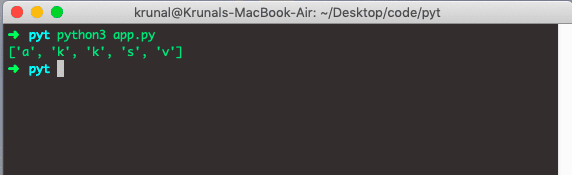See, we can see the sorted elements inside the list.

Now, let’s take a list with the integer items in it.

```# app.py

listB = [21, 19, 46, 18, 29]
print(sorted(listB))```

See the output.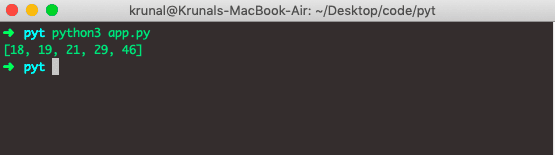## Let’s sort a string. See the following code.

```# app.py

strA = 'AppDividend'
print(sorted(strA))```

See the output.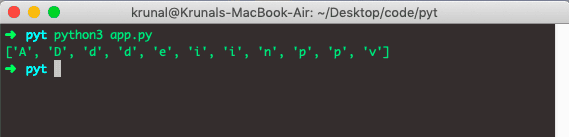Now, let’s take a list of strings and sort that list.

```# app.py

listStr = ['Venom', 'Toxin', 'Carnage', 'Knull']
print(sorted(listStr))```

See the output.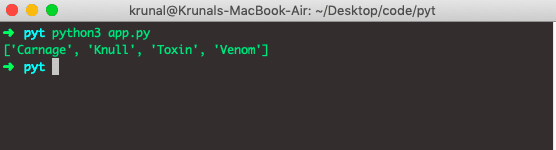It will return the sorted strings in a list.

## Add a key as a parameter in a Sorted Function

Let’s add a key as a parameter to the sorted() function.

```# app.py

statementA = 'We do whatever we want because We are Venom'
print(sorted(statementA.split(), key=str.upper))```

See the below.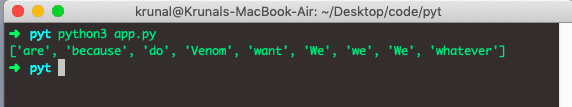Let’s add a reverse parameter to the Python Sorted function.

```# app.py

strB = 'Amazon'
print(sorted(strB, reverse=True))```

See the output.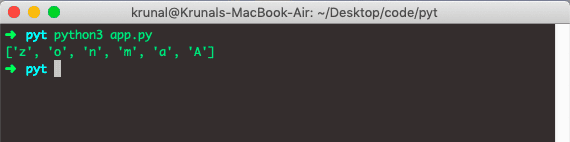## How to sort a dictionary in Python

To sort a dictionary in Python, use the sorted() method. The sorted() function returns a sorted dictionary from the given iterable.

Let’s sort a dictionary.

```# app.py

dictA = {
19: 'Beautiful',
21: 'Handsome',
18: 'Gorgeous',
46: 'Glamorous'
}
print(sorted(dictA, reverse=True))```

It will give us the output based on the dictionary keys.

See the output.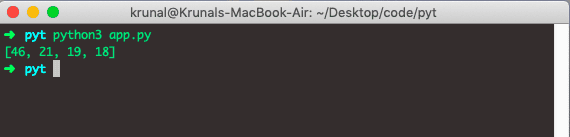## How to sort a tuple in Python

To sort a tuple in Python, use the sorted() method. The sorted() function returns a sorted tuple from the given iterable.

Let’s take the example of a Tuple.

```# app.py

tupA = ('Penny', 'Sheldon', 'Raj', 'Leonard')
print(sorted(tupA))```

It will output based on the Alphabetical order.

See the output.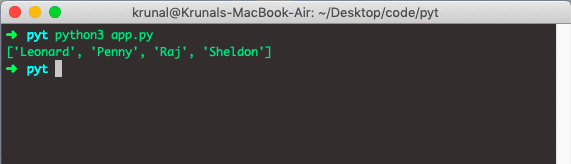That’s it for this tutorial.

This site uses Akismet to reduce spam. Learn how your comment data is processed.